# In your own words what do you think

• Slides: 44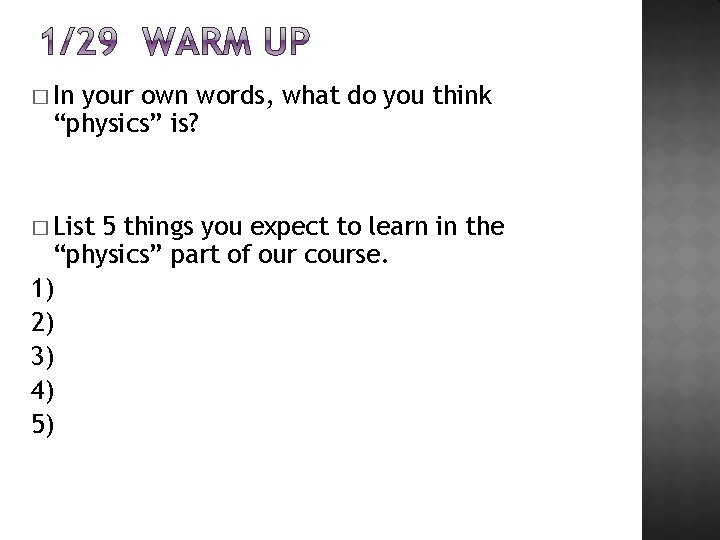� In your own words, what do you think “physics” is? � List 5 things you expect to learn in the “physics” part of our course. 1) 2) 3) 4) 5)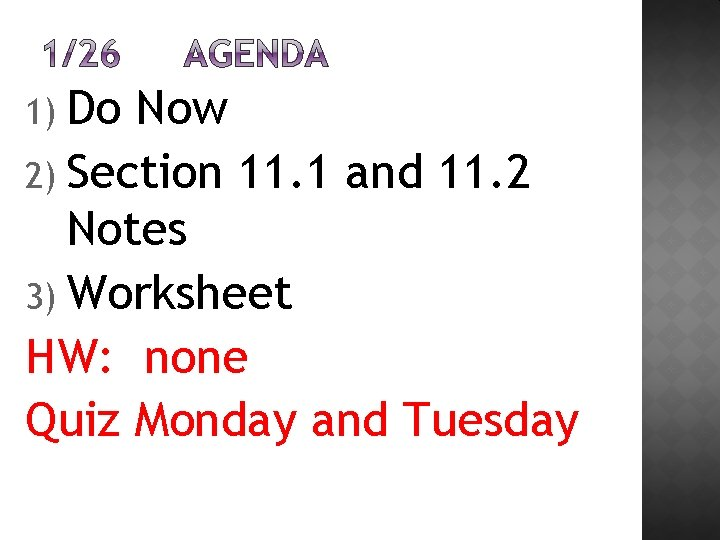1) Do Now 2) Section 11. 1 and 11. 2 Notes 3) Worksheet HW: none Quiz Monday and Tuesday� Were you surprised by your results from yesterday’s personality quiz? Explain Why or Why Not? � List 5 possible jobs or careers that are recommended or related to your personality type. 1) 2) 3) 4) 5)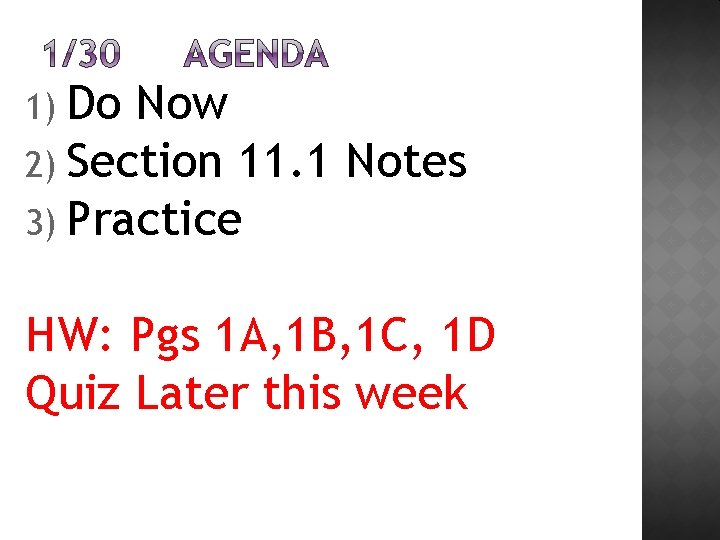1) Do Now 2) Section 11. 1 Notes 3) Practice HW: Pgs 1 A, 1 B, 1 C, 1 D Quiz Later this weekMotion� Choosing a frame of reference �To describe motion accurately and completely, a frame of reference is necessary. Ex: A butterfly moves, but so does the Earth. �Frame of reference-system of objects that are not moving with respect to one another. � How fast are you moving? �Relative Motion- Movement in relation to a frame of reference. Ex: A train to people on a platform is moving. To people on the train, they don’t seem to be moving.� Distance- The length of a path between two points. � Measuring Displacement �Displacement is the direction from the starting point and the length of a straight line from the starting point to the ending point. � Directions use displacements �Ex: Walk 5 blocks north. �Do Quick Lab on comparing distance and displacement � Walk around desks… tape measurer.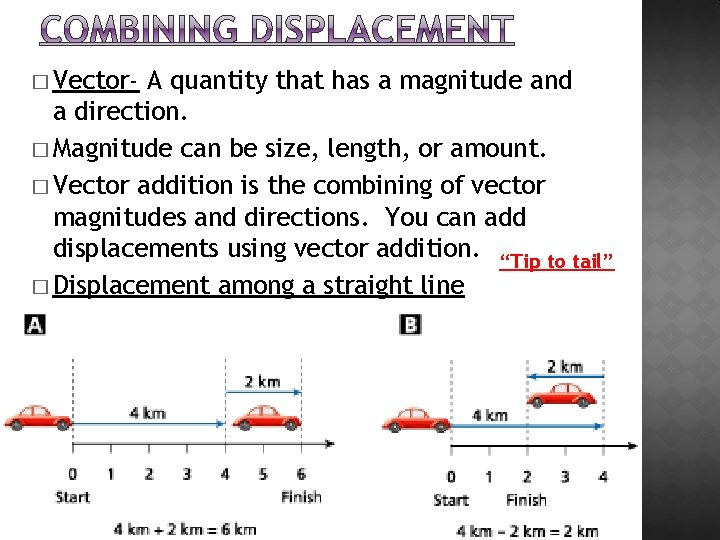� Vector- A quantity that has a magnitude and a direction. � Magnitude can be size, length, or amount. � Vector addition is the combining of vector magnitudes and directions. You can add displacements using vector addition. “Tip to tail” � Displacement among a straight line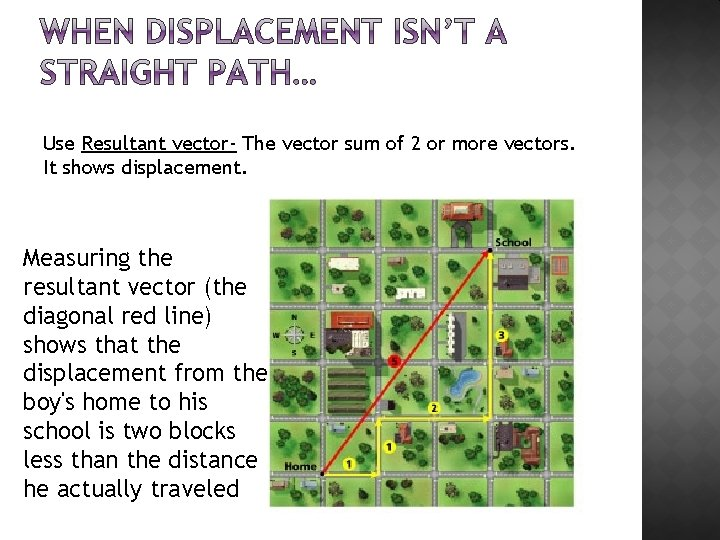Use Resultant vector- The vector sum of 2 or more vectors. It shows displacement. Measuring the resultant vector (the diagonal red line) shows that the displacement from the boy's home to his school is two blocks less than the distance he actually traveled� Your vacation to Miami requires a connecting flight. Your airplane first flies 760 miles West to Chicago, then 1400 mi south to Miami. How many miles would the trip have been without the connecting flight?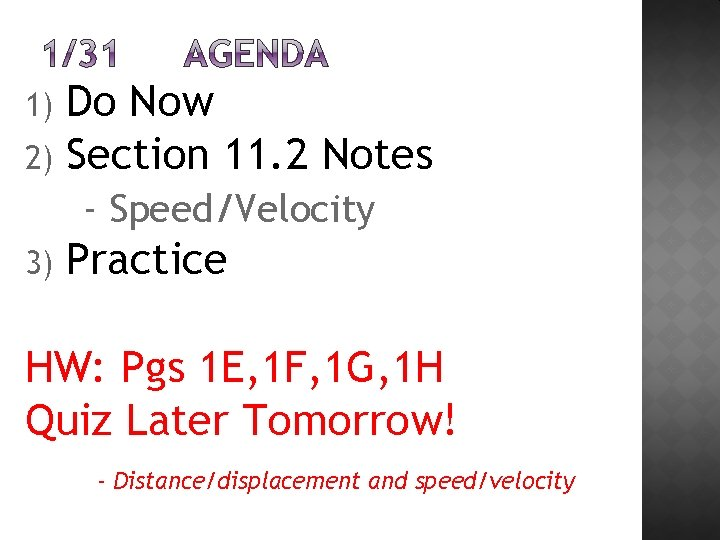Do Now 2) Section 11. 2 Notes 1) - Speed/Velocity 3) Practice HW: Pgs 1 E, 1 F, 1 G, 1 H Quiz Later Tomorrow! - Distance/displacement and speed/velocity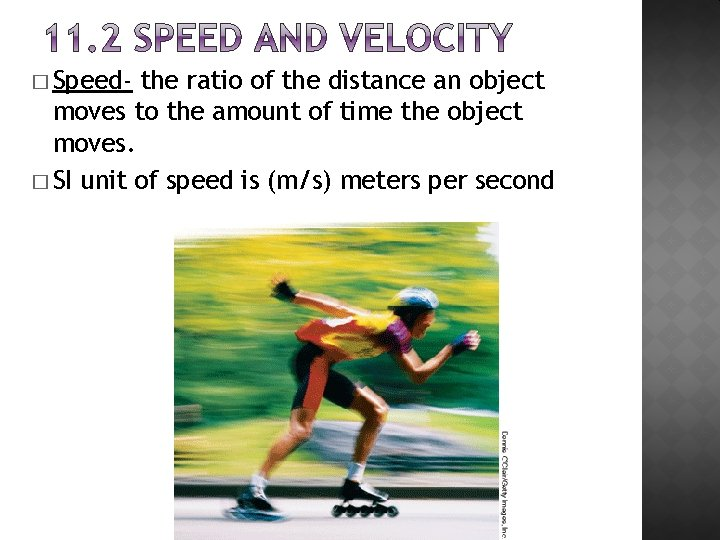� Speed- the ratio of the distance an object moves to the amount of time the object moves. � SI unit of speed is (m/s) meters per second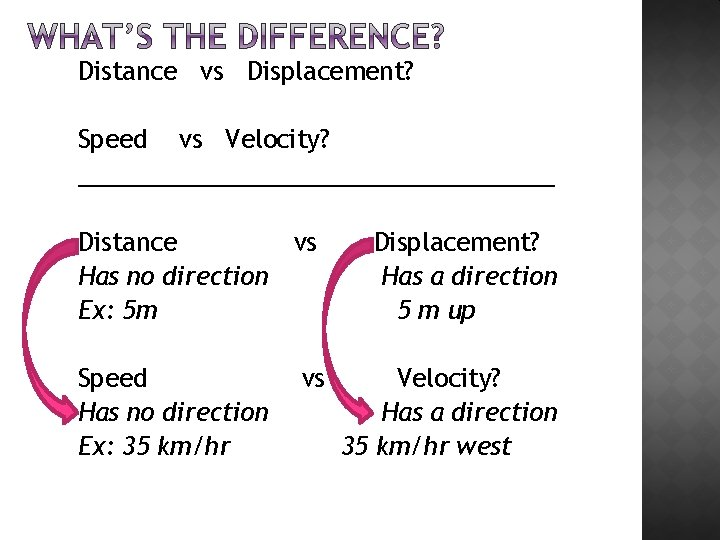Distance vs Displacement? Speed vs Velocity? __________________ Distance vs Has no direction Ex: 5 m Speed Has no direction Ex: 35 km/hr vs Displacement? Has a direction 5 m up Velocity? Has a direction 35 km/hr westInstantaneous speed- measured at a particular instant. Instantaneous Speed, v, the rate at which an object is moving at a given moment in time. Example: A car’s speedometer.Average speed- computed for the entire duration of a trip. �speed-, v, is the total distance traveled, d, divided by the time, t, it takes to travel that distance. Average speed = Total distance total time or v=d t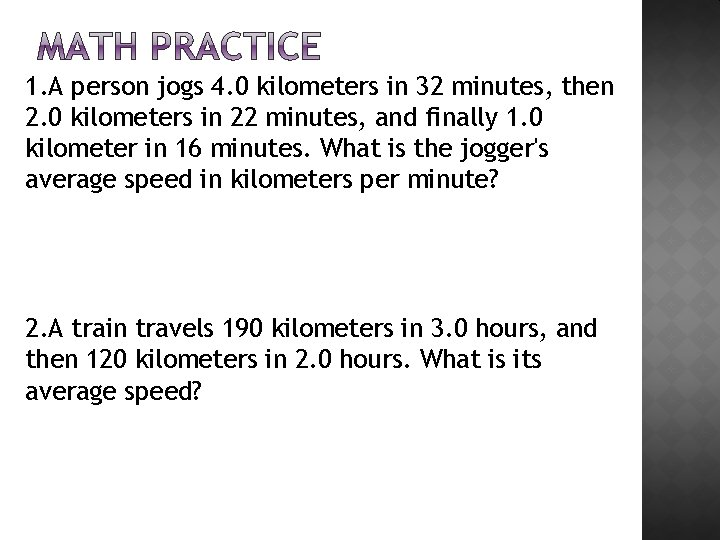1. A person jogs 4. 0 kilometers in 32 minutes, then 2. 0 kilometers in 22 minutes, and finally 1. 0 kilometer in 16 minutes. What is the jogger's average speed in kilometers per minute? 2. A train travels 190 kilometers in 3. 0 hours, and then 120 kilometers in 2. 0 hours. What is its average speed?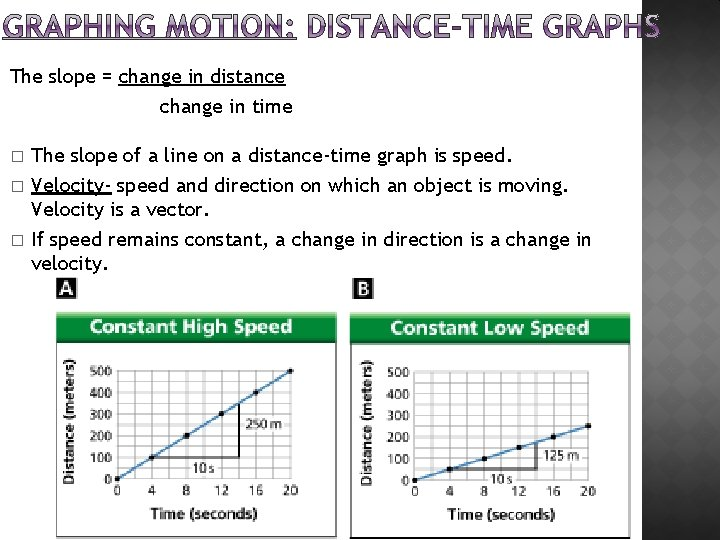The slope = change in distance change in time � � � The slope of a line on a distance-time graph is speed. Velocity- speed and direction on which an object is moving. Velocity is a vector. If speed remains constant, a change in direction is a change in velocity.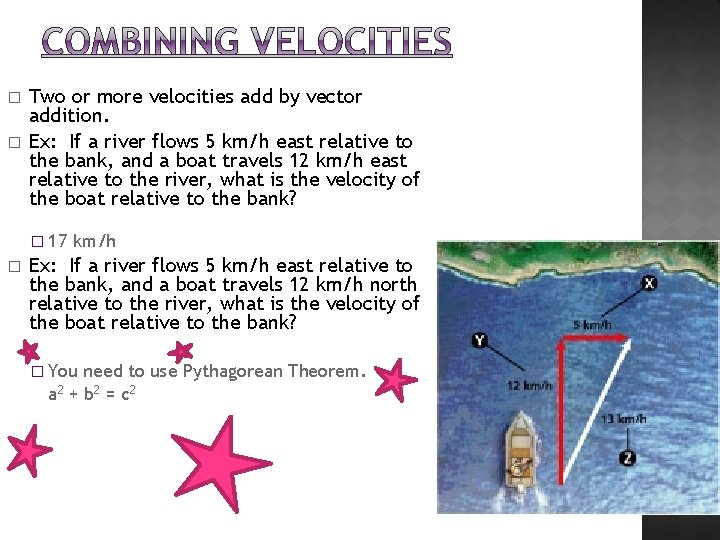� � Two or more velocities add by vector addition. Ex: If a river flows 5 km/h east relative to the bank, and a boat travels 12 km/h east relative to the river, what is the velocity of the boat relative to the bank? � 17 � km/h Ex: If a river flows 5 km/h east relative to the bank, and a boat travels 12 km/h north relative to the river, what is the velocity of the boat relative to the bank? � You need to use Pythagorean Theorem. a 2 + b 2 = c 2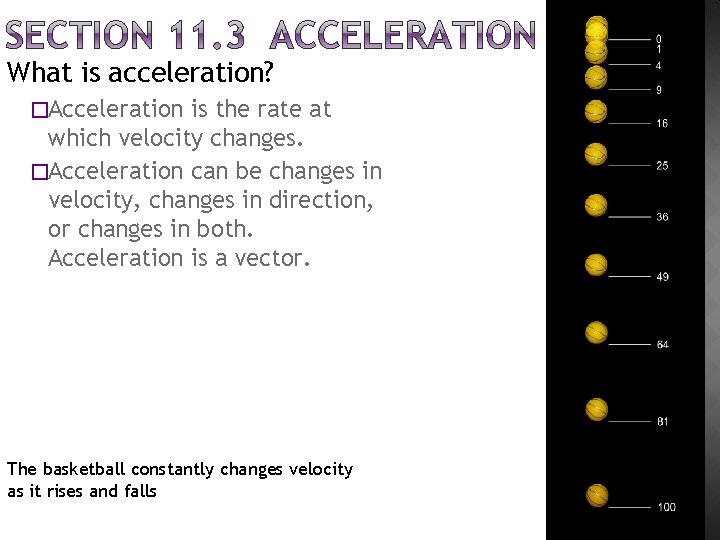What is acceleration? �Acceleration is the rate at which velocity changes. �Acceleration can be changes in velocity, changes in direction, or changes in both. Acceleration is a vector. The basketball constantly changes velocity as it rises and falls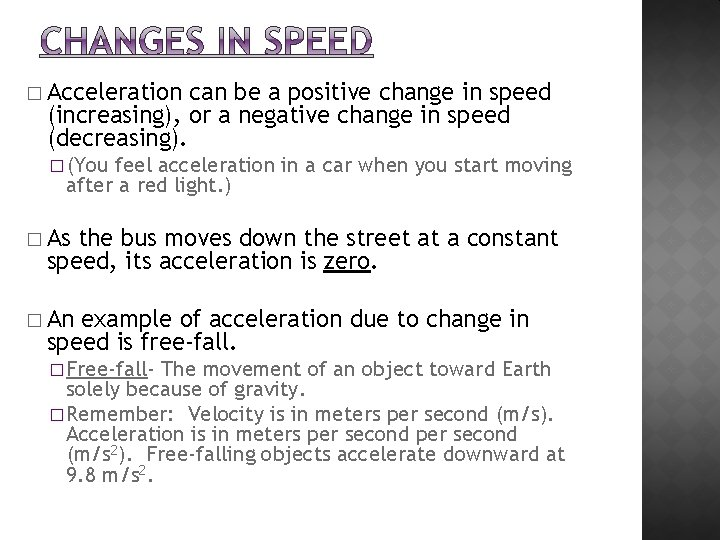� Acceleration can be a positive change in speed (increasing), or a negative change in speed (decreasing). � (You feel acceleration in a car when you start moving after a red light. ) � As the bus moves down the street at a constant speed, its acceleration is zero. � An example of acceleration due to change in speed is free-fall. � Free-fall- The movement of an object toward Earth solely because of gravity. � Remember: Velocity is in meters per second (m/s). Acceleration is in meters per second (m/s 2). Free-falling objects accelerate downward at 9. 8 m/s 2.� Constant Acceleration- A steady change in velocity. � Velocity second. � of the object changes by the same amount each Calculating Acceleration � You calculate acceleration for straight-line motion by dividing the change in velocity by the total time. a = acceleration t = total time vi = initial velocity a = change in velocity total time vf = final velocity a = vf - v i t Constant acceleration during takeoff results in changes to an aircraft's velocity that are in a constant direction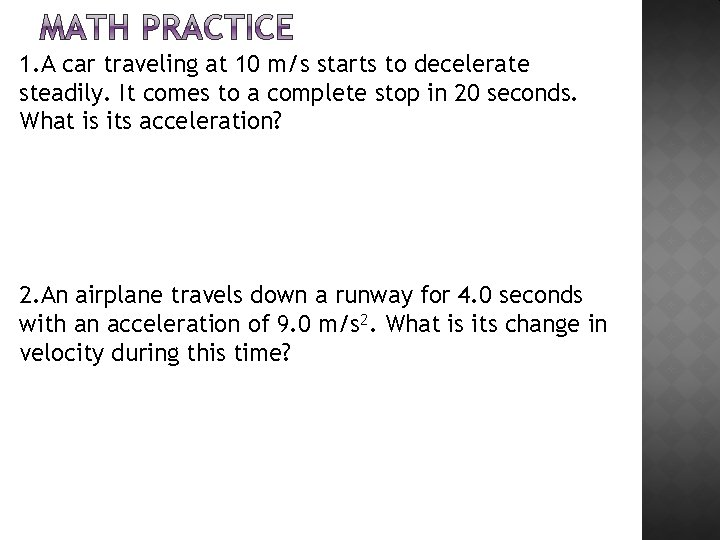1. A car traveling at 10 m/s starts to decelerate steadily. It comes to a complete stop in 20 seconds. What is its acceleration? 2. An airplane travels down a runway for 4. 0 seconds with an acceleration of 9. 0 m/s 2. What is its change in velocity during this time?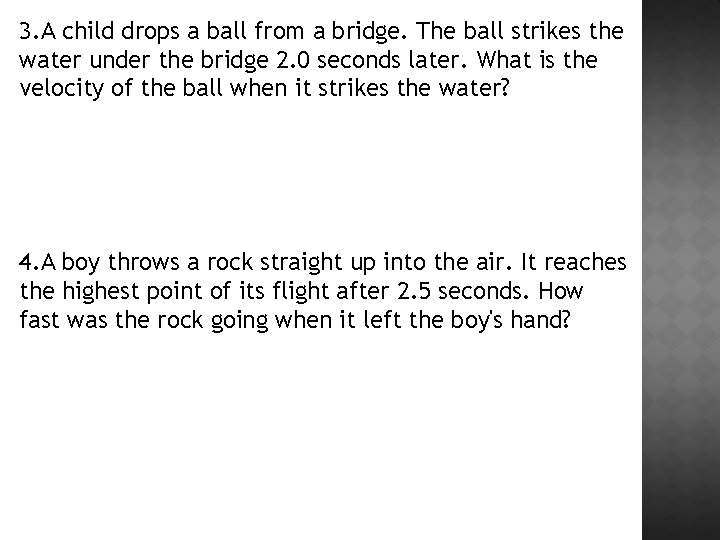3. A child drops a ball from a bridge. The ball strikes the water under the bridge 2. 0 seconds later. What is the velocity of the ball when it strikes the water? 4. A boy throws a rock straight up into the air. It reaches the highest point of its flight after 2. 5 seconds. How fast was the rock going when it left the boy's hand?5. A car is moving from rest and attained a velocity of 80 m/s. Calculate the acceleration of the car after 5 s? a = ΔV t a = Vf - Vi t a= Vf= Vi= t=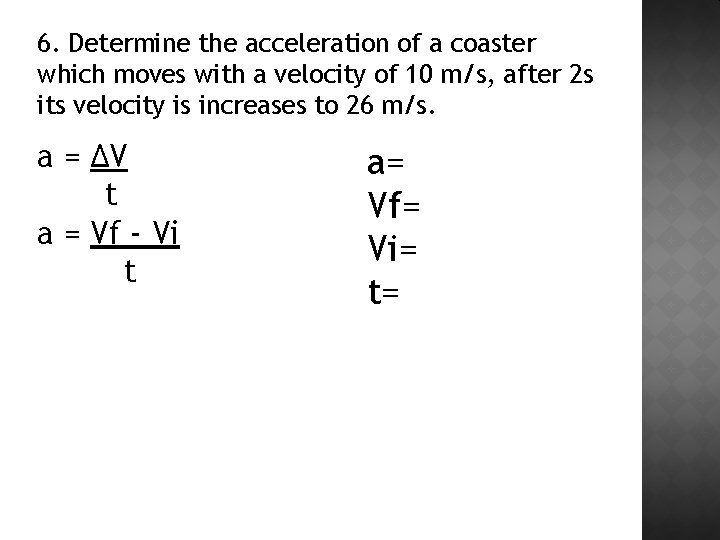6. Determine the acceleration of a coaster which moves with a velocity of 10 m/s, after 2 s its velocity is increases to 26 m/s. a = ΔV t a = Vf - Vi t a= Vf= Vi= t=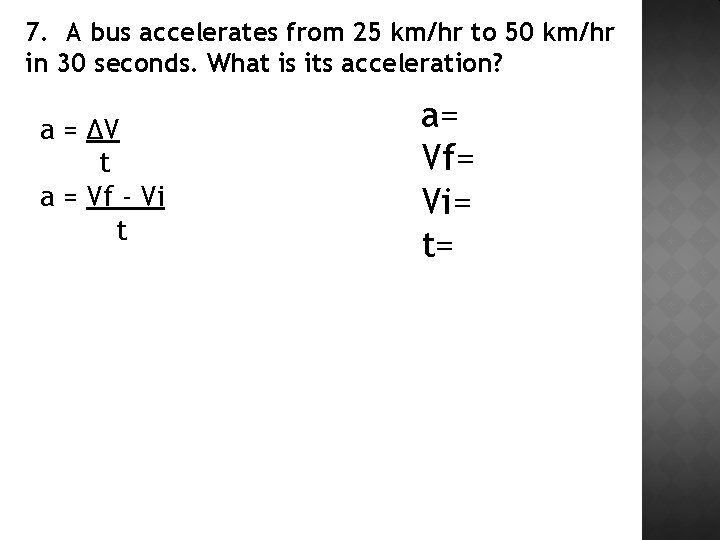7. A bus accelerates from 25 km/hr to 50 km/hr in 30 seconds. What is its acceleration? a = ΔV t a = Vf - Vi t a= Vf= Vi= t=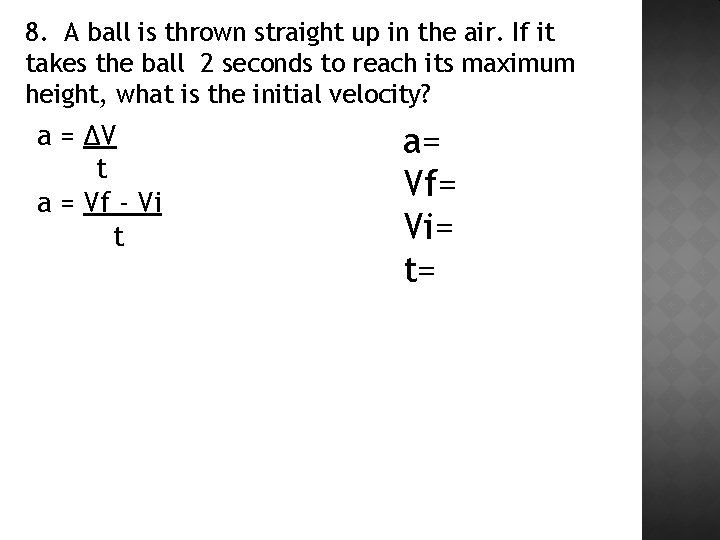8. A ball is thrown straight up in the air. If it takes the ball 2 seconds to reach its maximum height, what is the initial velocity? a = ΔV t a = Vf - Vi t a= Vf= Vi= t=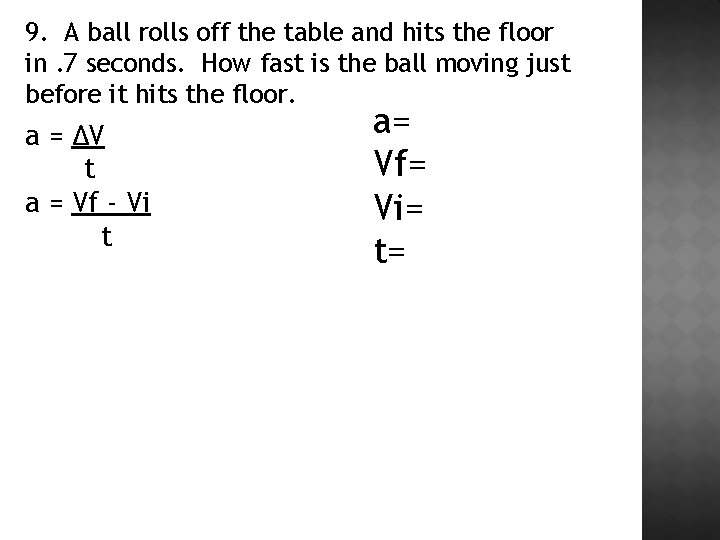9. A ball rolls off the table and hits the floor in. 7 seconds. How fast is the ball moving just before it hits the floor. a = ΔV t a = Vf - Vi t a= Vf= Vi= t=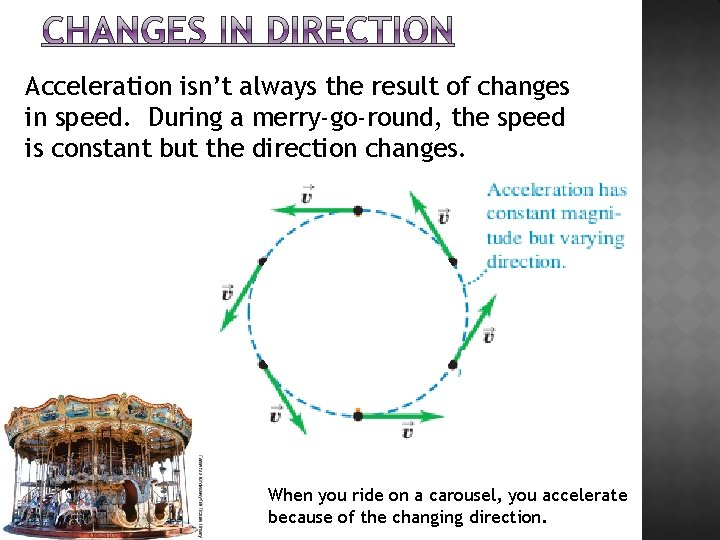Acceleration isn’t always the result of changes in speed. During a merry-go-round, the speed is constant but the direction changes. When you ride on a carousel, you accelerate because of the changing direction.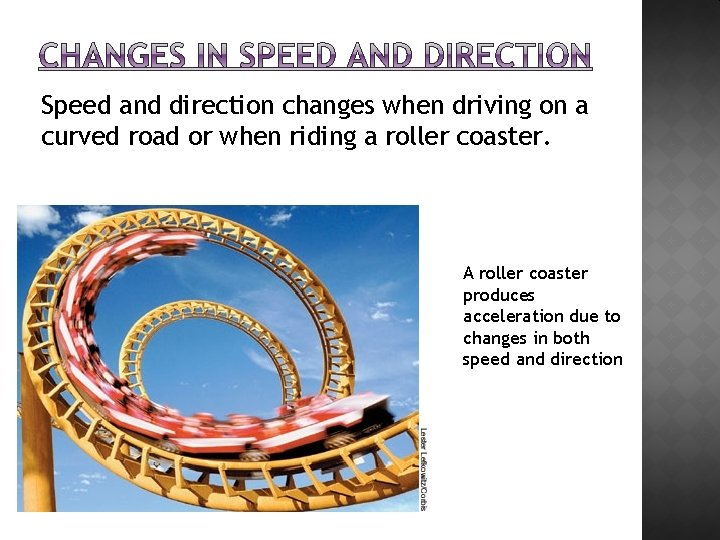Speed and direction changes when driving on a curved road or when riding a roller coaster. A roller coaster produces acceleration due to changes in both speed and direction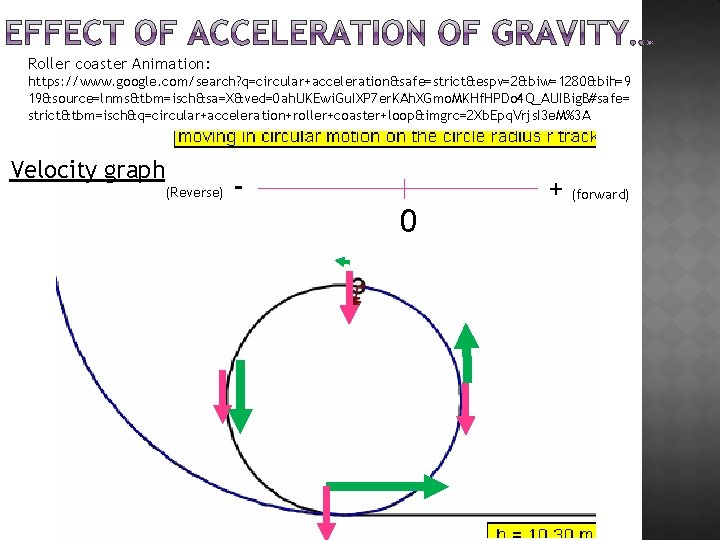Roller coaster Animation: https: //www. google. com/search? q=circular+acceleration&safe=strict&espv=2&biw=1280&bih=9 19&source=lnms&tbm=isch&sa=X&ved=0 ah. UKEwi. Gu. IXP 7 er. KAh. XGmo. MKHf. HPDo 4 Q_AUIBig. B#safe= strict&tbm=isch&q=circular+acceleration+roller+coaster+loop&imgrc=2 Xb. Epq. Vrjs. I 3 e. M%3 A Velocity graph (Reverse) - 0 + (forward)The slope of the speed-time graph is acceleration. �Speed-Time Graphs Linear graph- the displayed data form straight-line parts. Slope = acceleration.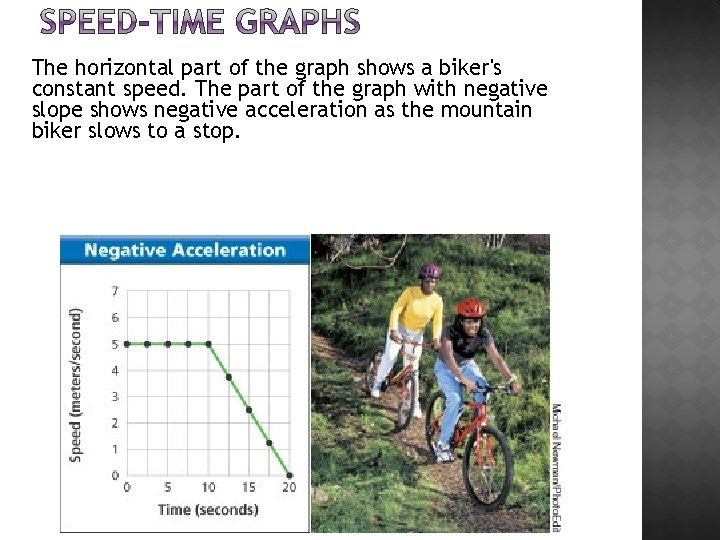The horizontal part of the graph shows a biker's constant speed. The part of the graph with negative slope shows negative acceleration as the mountain biker slows to a stop.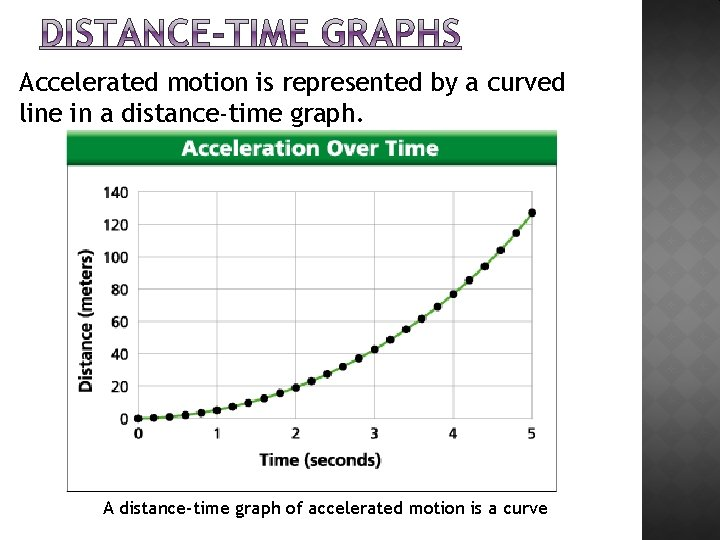Accelerated motion is represented by a curved line in a distance-time graph. A distance-time graph of accelerated motion is a curve1) Do Now 2) Finish Section 11. 1 and 11. 2 Notes 3) Worksheet HW: Pgs 1, 2 Quiz Monday and Tuesday1. What is the velocity of a car that traveled a total of 75 Km North in 1. 5 hrs? 2. What is the velocity of a plane that traveled 3000 miles from NY to CA in 5 hours?1) Do Now 2) Go over HW 3) Quiz 4) Graphing Practice pg 7, 8 5) HW: 3, 4, 7, 8, 10, 11, 16, 17 6) Quiz Wednesday - Math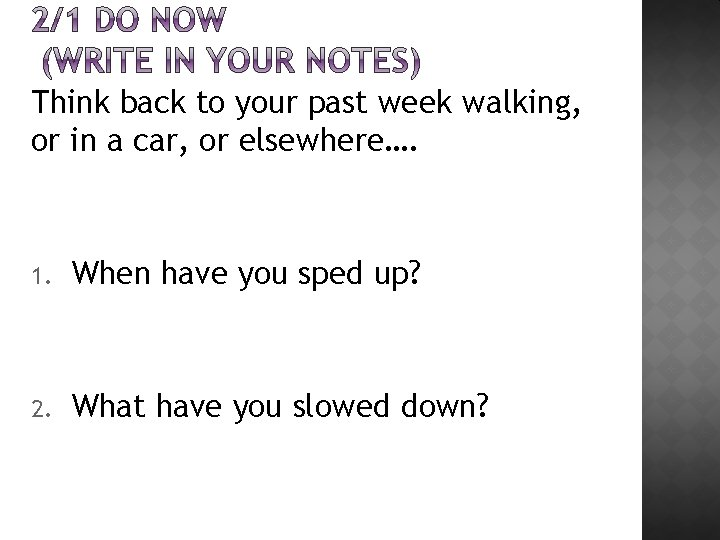Think back to your past week walking, or in a car, or elsewhere…. 1. When have you sped up? 2. What have you slowed down?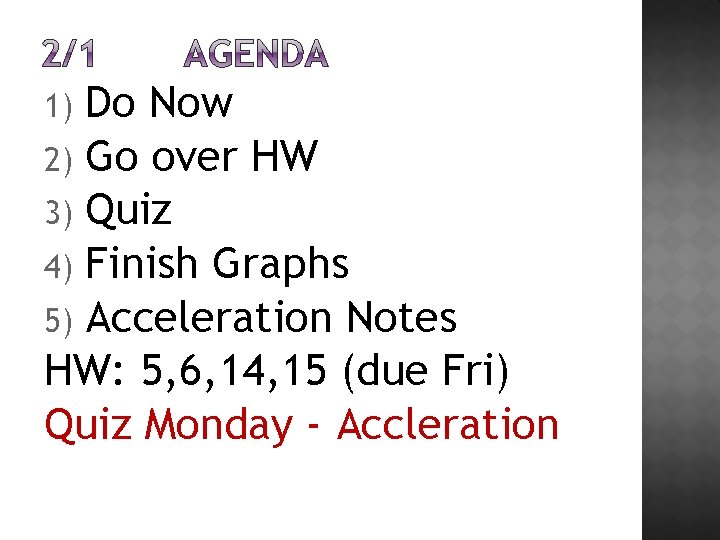Do Now 2) Go over HW 3) Quiz 4) Finish Graphs 5) Acceleration Notes HW: 5, 6, 14, 15 (due Fri) Quiz Monday - Accleration 1)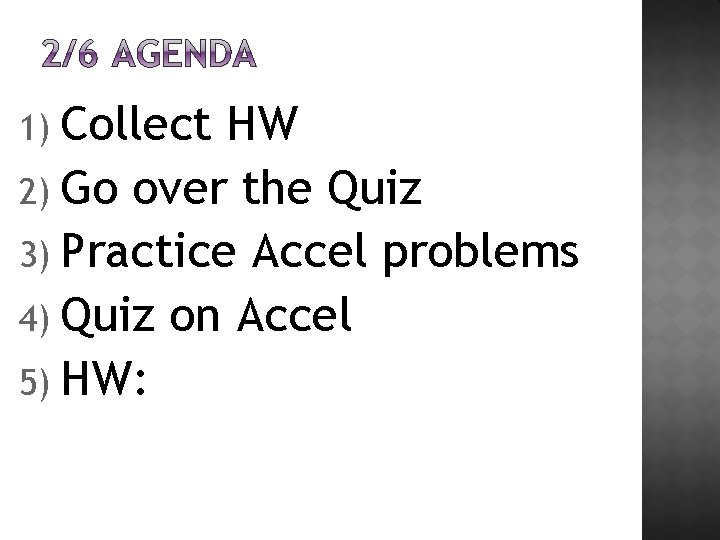1) Collect HW 2) Go over the Quiz 3) Practice Accel problems 4) Quiz on Accel 5) HW:1. A tennis ball thrown to the sky has what velocity as it reaches it’s highest point? 2. What is the acceleration of gravity on the ball at the highest point (give a #)? 3. What is the acceleration of gravity on the ball as it starts to fall back to earth (give a #)? ? 4. Which will have a higher acceleration of gravity: a tennis ball, or a bowling ball?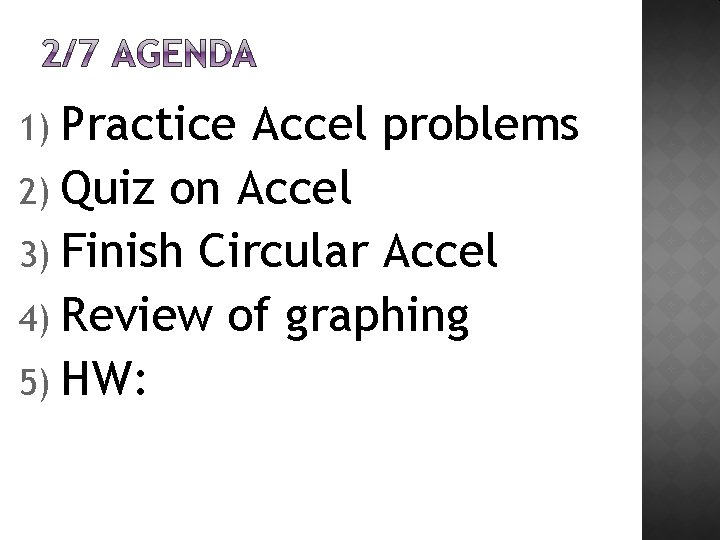1) Practice Accel problems 2) Quiz on Accel 3) Finish Circular Accel 4) Review of graphing 5) HW: